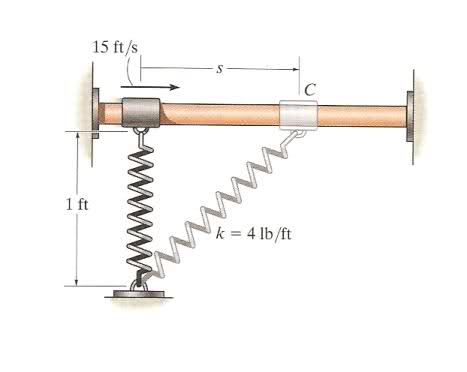# Kinetics, springs

red123

## Homework Statement

The 2-lb collar C fits loosely on the smooth shaft. If the spring is unstretched when s = 0 and the collar is given a velocity of 15 ft/s, determine the velocity of the collar when s = 1 ft.F = kx

## The Attempt at a Solution

x = l-l0 = (√[1 + s2] - 1) ft

Fsp = kx = [4√(1 + s2) - 4] lb

So, now I've found the spring force, how does velocity fit into this?

Last edited:

Kabbotta
Kinetic energy. Some has been given to the spring, whatever is left...

red123
Kinetic energy. Some has been given to the spring, whatever is left...

But how do I calculate the velocity?

Kabbotta
I guess I am a little surprised they gave you a question this hard without even explaining how to calculate kinetic energy.

Work-Kinetic Energy Thm.
$$K = 1/2mv^2$$
$$\sum W = K_{f} - K_{i} + W_{other forces}$$

The spring force is your other force doing work, and it will be negative work that takes away some kinetic energy, the final kinetic energy contains the velocity you are looking for, but the spring force is not constant so you have to do an integral for the work.

$$W_{spring} = \int F \cdot dx$$